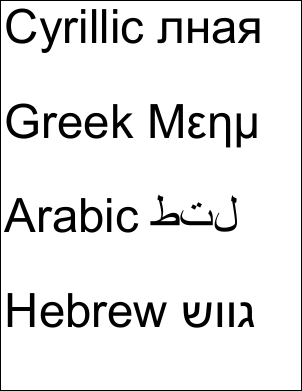eForm FDF ExampleThis example shows how to extract Unicode annotation values from an eForm FDF file.Src

 First we create an ABCpdf Doc object and read in our FDF file.   ```Doc theFDF = new Doc(); theFDF.Read(Server.MapPath("../Rez/form.fdf")); ``` ```Dim theFDF As New Doc() theFDF.Read(Server.MapPath("../Rez/form.fdf")) ```Dest

 We find out how many items there are in the FDF file and prepare to iterate through them.   ```string theValues = ""; int theLastID = Convert.ToInt32(theFDF.GetInfo(0, "Count")); ``` ```Dim theValues As String = "" Dim theLastID As Integer = Convert.ToInt32(theFDF.GetInfo(0, "Count")) ```We go through each item. We check to see if it is an annotation. If it is we check to see if the annotation type is text. If we have found a text annotation we extract the content and add the value to our list.   ```// extract annotation values (for insertion into PDF) for (int i = 0; i <= theLastID; i++) {   string theType = theFDF.GetInfo(i, "Type");   if (theType == "anno") {     if (theFDF.GetInfo(i, "SubType") == "Text") {       string theCont;       theCont = theFDF.GetInfo(i, "Contents");       theValues = theValues + theCont + "\r\n\r\n";     }   } } // extract field values (for demonstration purposes) for (int i = 0; i <= theLastID; i++) {   int theN = theFDF.GetInfoInt(i, "/FDF*/Fields*:Count");   for (int j = 0; j < theN; j++) {     string theName = theFDF.GetInfo(i, "/FDF*/Fields*[" + j + "]*/T:Text");     string theValue = theFDF.GetInfo(i, "/FDF*/Fields*[" + j + "]*/V:Text");     // here we would do something with the field value we've found   } } ``` ```' extract annotation values (for insertion into PDF) Dim i As Integer = 0 While i <= theLastID   Dim theType As String = theFDF.GetInfo(i, "Type")   If theType = "anno" Then     If theFDF.GetInfo(i, "SubType") = "Text" Then       Dim theCont As String       theCont = theFDF.GetInfo(i, "Contents")       theValues = theValues + theCont + vbCr & vbLf & vbCr & vbLf     End If   End If   System.Math.Max(System.Threading.Interlocked.Increment(i),i - 1) End While ' extract field values (for demonstration purposes) Dim i As Integer = 0 While i <= theLastID   Dim [theN] As Integer = theFDF.GetInfoInt(i, "/FDF*/Fields*:Count")   Dim j As Integer = 0   While j < [theN]     Dim theName As String = theFDF.GetInfo(i, "/FDF*/Fields*[" + j + "]*/T:Text")       ' here we would do something with the field value we've found     Dim theValue As String = theFDF.GetInfo(i, "/FDF*/Fields*[" + j + "]*/V:Text")     System.Math.Max(System.Threading.Interlocked.Increment(j),j - 1)   End While   System.Math.Max(System.Threading.Interlocked.Increment(i),i - 1) End While ```Finally we add the Unicode text to a new document and save it.   ```using (Doc doc = new Doc()) {   doc.Font = doc.EmbedFont("Arial", LanguageType.Unicode, false, true);   doc.FontSize = 96;   doc.Rect.Inset(10, 10);   doc.AddText(theValues);   doc.Save(Server.MapPath("fdf.pdf")); } ``` ```Using doc As New Doc()   doc.Font = doc.EmbedFont("Arial", LanguageType.Unicode, False, True)   doc.FontSize = 96   doc.Rect.Inset(10, 10)   doc.AddText(theValues)   doc.Save(Server.MapPath("fdf.pdf")) End Using ```This is the kind of PDF you might expect to produce.fdf.pdf